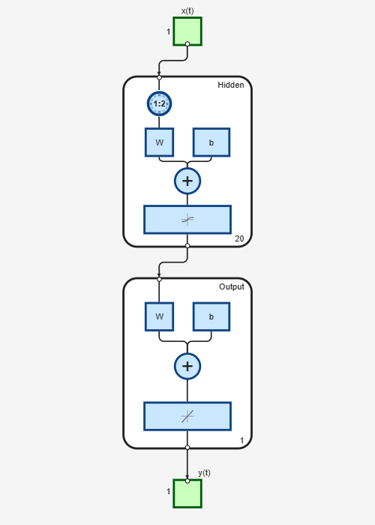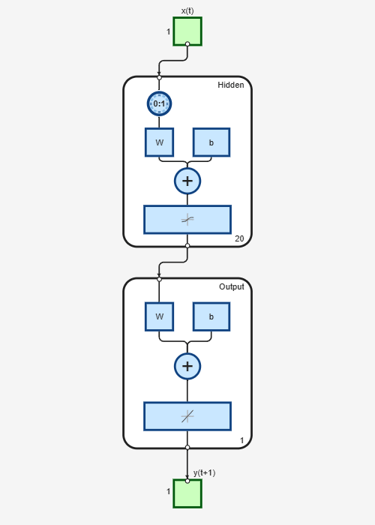# removedelay

Remove delay to neural network’s response

## Syntax

```net = removedelay(net,n) ```

## Description

`net = removedelay(net,n)` takes these arguments,

 `net` Neural network `n` Number of delays

and returns the network with input delay connections decreased, and output feedback delays increased, by the specified number of delays `n`. The result is a network which behaves identically, except that outputs are produced `n` timesteps earlier.

If the number of delays `n` is not specified, a default of one delay is used.

## Examples

### Remove and Add Delay to Network

This example creates, trains, and simulates a time delay network in its original form, on an input time series `X` and target series `T`. Then the delay is removed and later added back. The first and third outputs will be identical, while the second result will include a new prediction for the following step.

```[X,T] = simpleseries_dataset; net1 = timedelaynet(1:2,20); [Xs,Xi,Ai,Ts] = preparets(net1,X,T); net1 = train(net1,Xs,Ts,Xi); y1 = net1(Xs,Xi); view(net1) ``````net2 = removedelay(net1); [Xs,Xi,Ai,Ts] = preparets(net2,X,T); y2 = net2(Xs,Xi); view(net2) ``````net3 = adddelay(net2); [Xs,Xi,Ai,Ts] = preparets(net3,X,T); y3 = net3(Xs,Xi); view(net3) ```